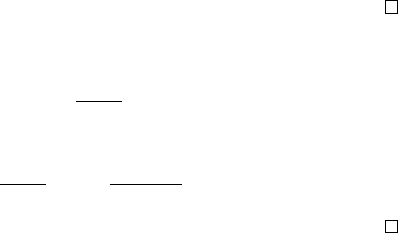4Matrices and linear equations

IA Vectors and Matrices4.2 Inverse of an n × n matrix
For larger matrices, the formula for the inverse is similar, but slightly more
complicated (and costly to evaluate). The key to finding the inverse is the
following:
Lemma.
P
A
ik
jk
= δ
ij
det A.
Proof.
If
i 6
=
j
, then consider an
n × n
matrix
B
, which is identical to
A
except
the
j
th row is replaced by the
i
th row of
A
. So
jk
of
B
= ∆
jk
of
A
, since
jk
does not depend on the elements in row
j
. Since
B
has a duplicate row, we know
that
0 = det B =
n
X
k=1
B
jk
jk
=
n
X
k=1
A
ik
jk
.
If i = j, then the expression is det A by the Laplace expansion formula.
Theorem. If det A 6= 0, then A
1
exists and is given by
(A
1
)
ij
=
ji
det A
.
Proof.
(A
1
)
ik
A
kj
=
ki
det A
A
kj
=
δ
ij
det A
det A
= δ
ij
.
So A
1
A = I.
The other direction is easy to prove. If
det A
= 0, then it has no inverse,
since for any matrix B, det AB = 0, and hence AB cannot be the identity.
Example.
Consider the shear matrix
S
λ
=
1 λ 0
0 1 0
0 0 1
. We have
det S
λ
= 1.
The cofactors are
11
= 1
12
= 0
13
= 0
21
λ
22
= 1
23
= 0
31
= 0
32
= 0
33
= 1
So S
1
λ
=
1 λ 0
0 1 0
0 0 1
.
How many arithmetic operations are involved in calculating the inverse of an
n × n matrix? We just count multiplication operations since they are the most
time-consuming. Suppose that calculating
det A
takes
f
n
multiplications. This
involves
n
(
n
1)
×
(
n
1) determinants, and you need
n
more multiplications to
put them together. So f
n
= nf
n1
+ n. So f
n
= O(n!) (in fact f
n
(1 + e)n!).
To find the inverse, we need to calculate
n
2
cofactors. Each is a
n
1
determinant, and each takes
O
((
n
1)!). So the time complexity is
O
(
n
2
(
n
1)!) =
O(n ·n!).
This is incredibly slow. Hence while it is theoretically possible to solve
systems of linear equations by inverting a matrix, sane people do not do so
in general. Instead, we develop certain better methods to solve the equations.
In fact, the “usual” method people use to solve equations by hand only has
complexity O(n
3
), which is a much better complexity.